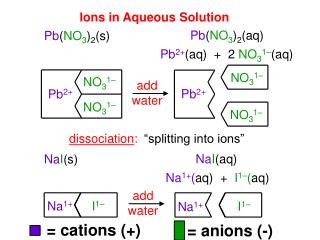DownloadDownload PresentationIons in Aqueous Solution

# Ions in Aqueous Solution

Télécharger la présentation## Ions in Aqueous Solution

- - - - - - - - - - - - - - - - - - - - - - - - - - - E N D - - - - - - - - - - - - - - - - - - - - - - - - - - -
##### Presentation Transcript

1. NO31– NO31– Pb2+ Pb2+ NO31– NO31– I1– Na1+ Na1+ I1– Ions in Aqueous Solution Pb(NO3)2(aq) Pb(NO3)2(s) Pb2+(aq) + 2 NO31–(aq) add water dissociation: “splitting into ions” NaI(aq) NaI(s) Na1+(aq) + I1–(aq) add water = cations (+) = anions (-)

2. _ FeCl3(aq) + _ Cu(NO3)2(aq) (?) (?) Fe3+ Cu2+ Cl1– NO31– For double-replacement rxns(or precipitate rxns), The reaction will occur if any product is: water a gas a precipitate driving forces Check new combinations to decide! = cations (+) = anions (-) 1 1 1 2 _ Pb(NO3)2(aq) + _ K2SO4(aq) _ PbSO4(s) + _ KNO3(aq) (?) (?) Pb2+ Pb2+ SO42- NO31– K1+ SO42- K1+ NO31– (ppt) (aq) NR Fe3+ Cu2+ Cl1– NO31– (aq) (aq)

3. precipitate: a solid product that forms in an aqueous reaction SPECTATOR IONS = cations (+) = anions (-) Na2CO3(aq) + Ca(NO3)2 (aq) CaCO3(s) + 2NaNO3(aq) 2Na1+(aq) + CO32–(aq) + Ca2+(aq) + 2NO31–(aq) COMPLETE IONIC EQUATION CaCO3(s) + 2Na1+ (aq) + 2NO31–(aq) CO32–(aq) + Ca2+(aq) CaCO3(s) NET IONIC EQUATION cloudy solution containing CaCO3(s) and NaNO3(aq) clear Ca(NO3)2 solution clear Na2CO3 solution

4. Cl1– clear ZnSO4 solution clear BaCl2 solution Ba2+ Cl1– Mix together ZnSO4(aq) and BaCl2(aq): ZnSO4(aq) BaCl2(aq) Zn2+(aq) + SO42-(aq) Ba2+(aq) + 2 Cl1–(aq) SO42- Zn2+

5. yields SO42- SO42- Cl1– Ba2+ Ba2+ Ba2+ Zn2+ Cl1– Mix them and get the complete ionic equation… 1 1 1 2 __Zn2+(aq) + __SO42–(aq) + __Ba2+(aq) + __Cl1–(aq) ppt 2 1 1 + __Zn2+(aq) + __Cl1-(aq) __BaSO4(s) Cancel spectator ions to get net ionic equation… 1 1 1 __Ba2+(aq) + __SO42–aq) __BaSO4(s) SO42-

6. In a reaction: atoms are rearranged mass AND are conserved energy charge Balancing Chemical Equations law of conservation of mass same # of atoms of each type on each side of equation =

7. Reaction Conditions and Terminology Certain symbols give important info about a reaction. (s) = solid (l) = liquid (g) = gas (aq) = aqueous (dissolved in H2O) NaCl(s) NaCl(aq) More on aqueous… -- “soluble” or “in solution” also indicate that a substance is dissolved in water (usually)

8. means... means ______ is added to the reaction MgCO3(s) MgO(s) + CO2(g) C6H5Cl + NaOH C6H5OH + NaCl C2H4(g) + H2(g) C2H6(g) Other symbols… (i.e., clues about the reaction) “yields” or “produces” heat Temp. at which we perform rxn. might be given. 400oC The catalyst used might be given. Pt

9. _ Ca + _ FeSO4 _ Pb + _ Al2O3 How do we know if a reaction will occur? Li K Ca Na Mg Al Zn Fe Pb H+ Cu Hg Ag Au For single-replacement rxns, use Activity Series. Elements above replace elements below. = cations (+) = anions (-) 1 1 1 1 _ Fe + _ CaSO4 No Reaction